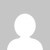# 18CS42 Design and Analysis of Algorithms Notes

[wptelegram-join-channel]

### 18CS42 Design and Analysis of Algorithms Notes

Here you can download the VTU CBCS 2018 Scheme notes, Question papers, and Study materials of 18CS42 Design and Analysis of Algorithms.

Important Topics Covered

What is an Algorithm? Algorithm Specification, Analysis Framework. Performance Analysis such as Space complexity, Time complexity. Asymptotic Notations such as Big-Oh notation (O), Omega notation (?), Theta notation (?), and Little-oh notation (o).

Mathematical analysis of Non-Recursive and recursive Algorithms with Examples. Important Problem Types: Sorting, Searching, String processing, Graph Problems, Combinatorial Problems. Fundamental Data Structures, Stacks, Queues, Graphs, Trees, Sets, and Dictionaries.

Divide and Conquer,  A general method, Binary search, Recurrence equation for divide and conquer, Finding the maximum and minimum. Merge sort, Quicksort, Strassen’s matrix multiplication. Advantages and Disadvantages of divide and conquer.

Greedy Method:General method, Coin Change Problem, Knapsack Problem, Job sequencing with deadlines. Optimal Tree problem and Transform and Conquer Approach.

The general method with Examples, Multistage Graphs of Dynamic Programming. Floyd’s Algorithm, Optimal Binary Search Trees, Knapsack problem. Backtracking, Branch and Bound, and NP-Complete and NP-Hard problems.

Click the below link to download the 2018 Scheme VTU CBCS Notes of 18CS42 Design and Analysis of Algorithms

M-1, M-2, M-3, M-4, and M-5 Another Set M-1, M-2, M-3, M-4, and M-5

Click the below link to download the 2018 Scheme VTU Question Papers of 18CS42 Design and Analysis of Algorithms

1.